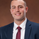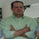3504 views
The Herrick Payoff Index is designed to show the amount of money flowing into or out of a futures contract. The Index uses open interest during its calculations, therefore, the security being analyzed must contain open interest.

The Herrick Payoff Index was developed by John Herrick.When the Herrick Payoff Index is above zero, it shows that money is flowing into the futures contract (which is bullish ). When the Index is below zero, it shows that money is flowing out of the futures contract (which is bearish ).

The interpretation of the Herrick Payoff Index involves looking for divergences between the Index and prices.
Open-source script

In true TradingView spirit, the author of this script has published it open-source, so traders can understand and verify it. Cheers to the author! You may use it for free, but reuse of this code in a publication is governed by House Rules. You can favorite it to use it on a chart.

Want to use this script on a chart?
```////////////////////////////////////////////////////////////
//  Copyright by shardison v1.0 08/05/2015
// Credit to GREENY for his work on the COT OPEN INTEREST SCRIPT
// Attention:
//The Herrick Payoff Index (HPI) was developed by John Herrick and is the only well known indicator that uses price, volume, and open interest.
// The Herrick Payoff Index is designed to show the amount of money flowing into or out of a futures contract.
//The Index uses open interest during its calculations, therefore, the security being analyzed must contain open interest.
//NOTE: THE COT OPEN INTEREST SCRIPT ONLY pulls Weekly DATA, hence only utilize this script on Weekly bars.
//Interpretation
//
//When the Herrick Payoff Index is above zero, it shows that money is flowing into the futures contract (which is bullish).
//When the Index is below zero, it shows that money is flowing out of the futures contract (which is bearish).
//
//The interpretation of the Herrick Payoff Index involves looking for divergences between the Index and prices.
////////////////////////////////////////////////////////////

study(title="Herrick Payoff Index", shorttitle="HPI")
valueofonecentmove = input(100, minval=1)
multiplyingfactor = input(10, minval=1)
wmaperiod = input(21, minval=1)
force_root = input("", title="Override Product")
is_includeoptions = input(false, type=bool, title="Include Options")

fxroot =
ticker == "USDCAD" ? "CD" :
ticker == "USDCAD" ? "CD" :
ticker == "USDCHF" ? "SF" :
ticker == "USDCZK" ? "CZ" :
ticker == "USDHUF" ? "FR" :
ticker == "USDILS" ? "IS" :
ticker == "USDJPY" ? "JY" :
ticker == "USDMXN" ? "MP" :
ticker == "USDNOK" ? "UN" :
ticker == "USDPLN" ? "PZ" :
ticker == "USDRUB" ? "RU" :
ticker == "USDSEK" ? "SE" :
ticker == "USDZAR" ? "RA" :
ticker == "EURUSD" ? "EC" :
ticker == "AUDUSD" ? "AD" :
ticker == "GBPUSD" ? "BP" :
ticker == "NZDUSD" ? "NE" :
ticker == "BRLUSD" ? "BR" :
""

is_inversed =
ticker == "USDCAD" ? true :
ticker == "USDCAD" ? true :
ticker == "USDCHF" ? true :
ticker == "USDCZK" ? true :
ticker == "USDHUF" ? true :
ticker == "USDILS" ? true :
ticker == "USDJPY" ? true :
ticker == "USDMXN" ? true :
ticker == "USDNOK" ? true :
ticker == "USDPLN" ? true :
ticker == "USDRUB" ? true :
ticker == "USDSEK" ? true :
ticker == "USDZAR" ? true :
false

root = force_root == "" ? fxroot == "" ? syminfo.root : fxroot : force_root
code = root + (is_includeoptions ? "_FO" : "_F") + "_L_ALL"

oi = security("QUANDL:CFTC/"+code+"|0", "D", close)

commercial_long_total = security("QUANDL:CFTC/"+code+"|4", "W", close)
commercial_short_total = security("QUANDL:CFTC/"+code+"|5", "W", close)
long_total = security("QUANDL:CFTC/"+code+"|1", "W", close)
short_total = security("QUANDL:CFTC/"+code+"|2", "W", close)

commercial_long = is_inversed ? commercial_short_total : commercial_long_total
commercial_short = is_inversed ? commercial_long_total : commercial_short_total
long = is_inversed ? short_total : long_total
short = is_inversed ? long_total : short_total

diff = commercial_long - commercial_short
cdiff = diff - diff
cI = abs(cdiff)
cG = max(diff, diff)
diff2 = long - short
openinterestdiff = oi - oi
I = abs(openinterestdiff)
G = max(oi, oi)
S = multiplyingfactor
C = valueofonecentmove
V = volume
M = (high + low) / 2
My = M
K1 = (C*V*(M - My))*(1 + ((2 * I)/(G)))
K2 = (C*V*(M - My))*(1 - ((2 * I)/(G)))
K = M > My ? K1 : K2
Ky = K
HPI = ((Ky +(K - Ky)) * S)/100000
HPI_Index = 100 * (HPI - lowest(HPI,100))/(highest(HPI,100) - lowest(HPI,100))
wma = wma(HPI, wmaperiod)
plot(HPI, color=green, title="HPI")
plot(HPI_Index, color=aqua, title="HPI Index-Turn off all others")
plot (wma, color=orange, title="HPI Weighted Moving Average")
plot (diff, color=red, title="Commercials Net-Position")
plot (diff2, color=blue, title="Non-Commercials Net-Position")
hline(0, color=gray, title="Zero", linestyle=dashed)
//plot(oi, color = gray, title="OI")```